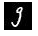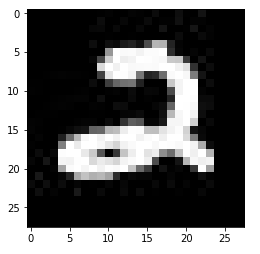# Get the filenames of the data in the Test set in the order they're predicted

I have an `ImageDataBunch` as shown

``````ImageDataBunch;

Train: LabelList (14000 items)
x: ImageList
Image (3, 32, 32),Image (3, 32, 32),Image (3, 32, 32),Image (3, 32, 32),Image (3, 32, 32)
y: CategoryList
1,1,1,1,1
Path: .;

Valid: LabelList (3500 items)
x: ImageList
Image (3, 32, 32),Image (3, 32, 32),Image (3, 32, 32),Image (3, 32, 32),Image (3, 32, 32)
y: CategoryList
1,1,1,1,0
Path: .;

Test: LabelList (4000 items)
x: ImageList
Image (3, 32, 32),Image (3, 32, 32),Image (3, 32, 32),Image (3, 32, 32),Image (3, 32, 32)
y: EmptyLabelList
,,,,
Path: .
``````

How do I get the file names of the objects in the test set in the order they’re predicted in?

2 Likes

Following worked for me. There might be easier ways to do this though

``````num = len(learn.data.test_ds)

for i in range(num):
filename = str(learn.data.test_ds.items[i]).split('/')[-1]
learn.predict(learn.data.test_ds[i])``````
4 Likes

@sumeetd Thank you for providing this! I used it to make something I could call.

``````datasetIndex = []
num = len(learn.data.test_ds)

for i in range(num):
datasetIndex.append(str(learn.data.test_ds.items[i]).split('/')[-1])``````
2 Likes

I have been struggling with this myself, I find it so frustrating that there’s no proper documentation on this, and it is completely unintuitive. Why can’t you just use .get_preds() and zip with the filenames…

2 Likes

The predictions are made in the order the files are fed to the DataBunch. For example, if you load the TestData from a DataFrame, the `i`th prediction will correspond with the `i`th entry in the dataframe (This is something I figured out from experimentation).

I believe the same happens when you use from_folder. The predictions are in the same order as the files are listed when perform `os.listdir` (I haven’t experimented with this though)

I’ve spent > 8 hours tracking this down. Why aren’t the predictions in the order of the test set?

``````import fastai
print(fastai.__version__)

1.0.59
``````

I have a MNIST dataset in the proper folder format

``````train/
0/
1/
2/
:
:
9/
valid/
0/
1/
2/
:
:
9/
test/
testimg_00000.jpg
testimg_00001.jpg
:
:
testimg_27999.jpg
``````

Using the preferred `data_block` API

``````path = Path('/kaggle/working/data')
data = (ImageList.from_folder(path)
.split_by_folder()
.label_from_folder()
.add_test_folder(path/'test')
.databunch())

data
``````

gives

``````ImageDataBunch;

Train: LabelList (39900 items)
x: ImageList
Image (3, 28, 28),Image (3, 28, 28),Image (3, 28, 28),Image (3, 28, 28),Image (3, 28, 28)
y: CategoryList
4,4,4,4,4
Path: /kaggle/working/data;

Valid: LabelList (2100 items)
x: ImageList
Image (3, 28, 28),Image (3, 28, 28),Image (3, 28, 28),Image (3, 28, 28),Image (3, 28, 28)
y: CategoryList
4,4,4,4,4
Path: /kaggle/working/data;

Test: LabelList (28000 items)
x: ImageList
Image (3, 28, 28),Image (3, 28, 28),Image (3, 28, 28),Image (3, 28, 28),Image (3, 28, 28)
y: EmptyLabelList
,,,,
Path: /kaggle/working/data
``````

The item counts indicate that all is good! However, when I try to predict from a learner, it is evident that the test set is not ordered correctly. This can be seen by indexing the test set:

``````data.test_ds.x
``````and then

``````i = 0
img = imageio.imread(str(f'/kaggle/working/data/test/testimg_{str(i).zfill(5)}.jpg'))
plt.imshow(img);
``````Why would these not be the same image? The file creation dates are in the order of the filenames. They are just created with:

``````for i in range(len(test)):
imageio.imsave(str(path / f'testimg_{str(i).zfill(5)}.jpg'), test[i])
``````

I was wondering why my validation accuracy was 0.99+ but the Kaggle leaderboard score of the submission was 0.10… The test order is “randomly” scrambled. I confirm that it’s correct above that

If

• you need to predict the test set from a learner in the order of a Kaggle submission file
• your test files alphabetical in the proper order (for example, are of the form `f'testimg_{str(i).zfill(5)}.jpg'` like `testimg_00133.jpg`)

this will do the trick:

``````preds, _ = learn.get_preds(ds_type=DatasetType.Test)
labels = np.argmax(preds, 1)
test_index = []
num = len(learn.data.test_ds)
for i in range(num):
test_index.append(str(learn.data.test_ds.items[i]).split('/')[-1])

df = (pd.DataFrame(data={"Label": labels, "Filename": test_index})
.sort_values(by='Filename')
.drop('Filename', axis=1)
.assign(ImageId = range(1, len(labels) + 1))
.reset_index(drop=True))[['ImageId', 'Label']]
``````
2 Likes

updated for fastai2

``````preds, targs = learn.get_preds()
filenames = []
for i in range(len(learn.dls.valid_ds)):
filenames.append(str(learn.dls.valid_ds.items[i]).split('/')[-1])
preds = [x.item() for x in preds]
df = pd.DataFrame(data={"prediction": preds, "target": targs, "filename": filenames})``````

Hi,
I am new to fastai, I will be thankful if you could help me to find a solution for this problem. I am trying to use segmentation for a data collection with 987 images, and I want to export my predicted images with the name of their real image(used as input in test file)? I tried to use this solution but I do not have any test_ds.

This is the code that I used for predicting:
dl = learn.dls.test_dl(fnames[:])
preds = learn.get_preds(dl=dl,reorder=False)
for i, pred in enumerate(preds):
pred_arg = pred.argmax(dim=0).numpy()
rescaled = pred_arg.astype(np.uint8)
im = Image.fromarray(rescaled)
im.save(f’/content/drive/MyDrive/classification/CNN_segmentation/big_Image/pred_all_tiff’)
I have posted question and searched to find the solution for this problem, but there is no resource for this problem.

Hi all,
I am facing the index 0 is out of bounds for axis 0 with size 0 error. My dataset structure is like this. In my dataset I have an audio folder, two subfolders (train folder) and (valid folder) are inside the audio folder. The train folder contains 7780 audio files while the valid folder contains 796 audio files. I tried each folder structure but every time facing the same error.